# please try to answer using the equations or explaining how to find each part    thank...

please try to answer using the equations or explaining how to find each part
thank u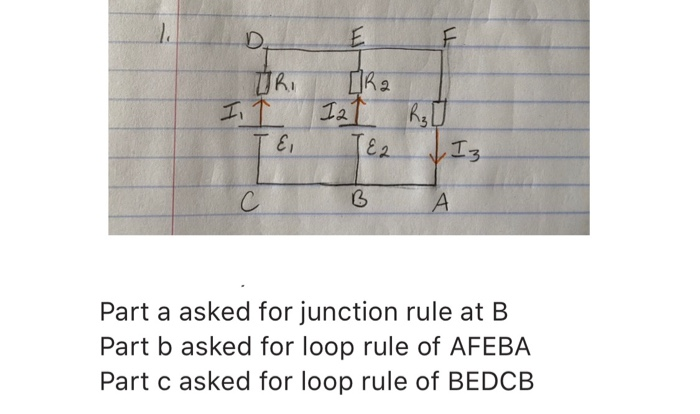و 1 D. E JRI IR IT I2 Τε, R30 TE2 Iz С B A Part a asked for junction rule at B Part b asked for loop rule of AFEBA Part c asked for loop rule of BEDCB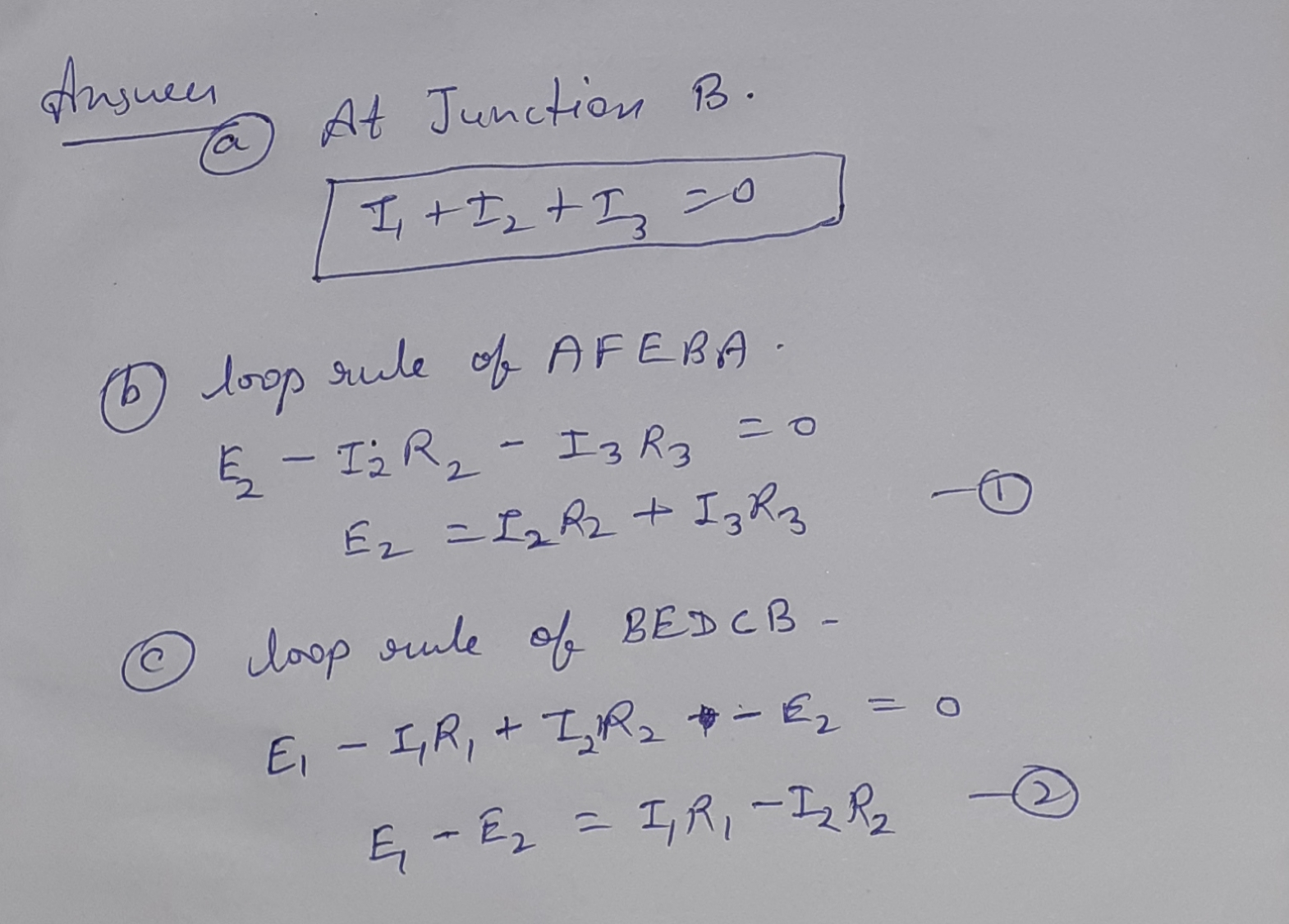Similar Homework Help Questions
• ### 1. For the circuit shown in the figure, we wish to find the currents 11, 12,...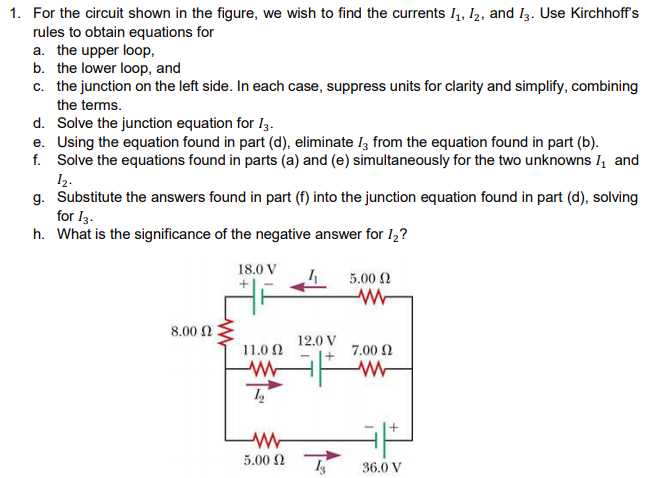1. For the circuit shown in the figure, we wish to find the currents 11, 12, and 13. Use Kirchhoffs rules to obtain equations for a. the upper loop, b. the lower loop, and c. the junction on the left side. In each case, suppress units for clarity and simplify, combining the terms. d. Solve the junction equation for Iz. e. Using the equation found in part (d), eliminate 13 from the equation found in part (b). f. Solve the...

• ### ANSWER ALL PLEASE Question 20 2 pts In the Figure below, V1 = 14 V, V2...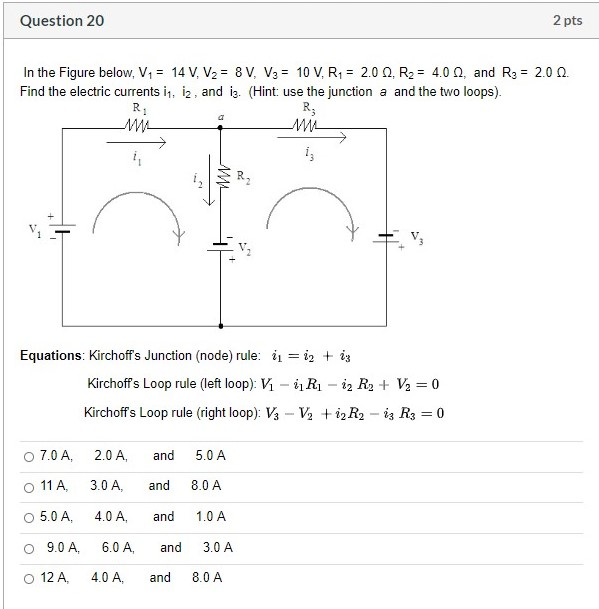ANSWER ALL PLEASE Question 20 2 pts In the Figure below, V1 = 14 V, V2 = 8 V, V3 = 10 V, R1 = 2.00, R2 = 4.0, and R3 = 2.00 Find the electric currents is, iz, and is. (Hint: use the junction a and the two loops). RI R 14 M4 Equations: Kirchoff's Junction (node) rule: 11 = 12 + iz Kirchoff's Loop rule (left loop): V1 - iRi - iz R2 + V2 = 0 Kirchoffs...

• ### please help with problems 7 and 8, I am extremely confused! Part 2: Kirchhoff's Rules For...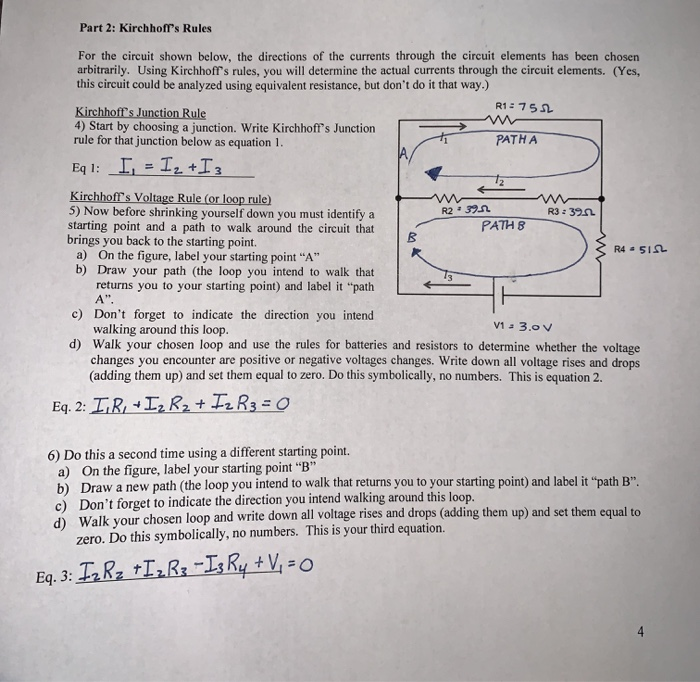please help with problems 7 and 8, I am extremely confused! Part 2: Kirchhoff's Rules For the circuit shown below, the directions of the currents through the circuit elements has been chosen arbitrarily. Using Kirchhoff's rules, you will determine the actual currents through the circuit elements. (Yes, this circuit could be analyzed using equivalent resistance, but don't do it that way.) R1: 752 Kirchhoff's Junction Rule 4) Start by choosing a junction. Write Kirchhoft's Junction rule for that junction below...

• ### Need help with all please!! Two charges are located along the x axis as shown below....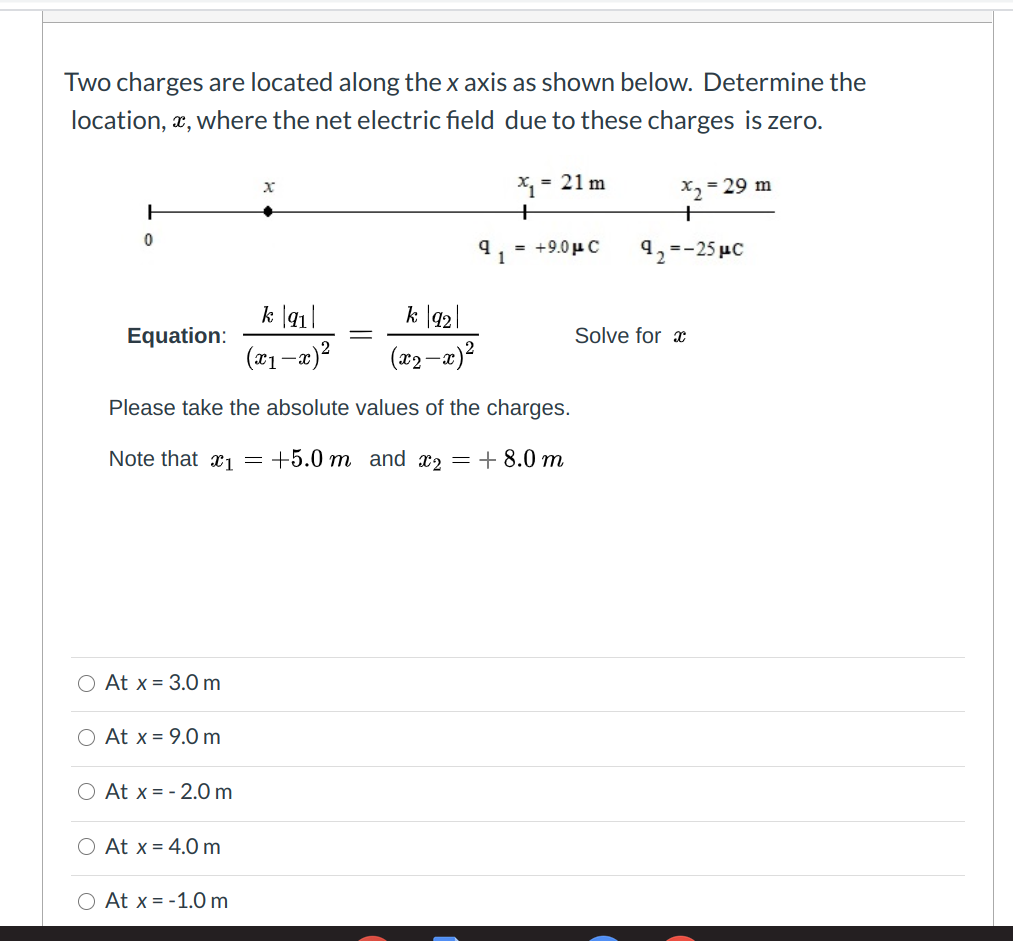Need help with all please!! Two charges are located along the x axis as shown below. Determine the location, X, where the net electric field due to these charges is zero. х *1 = 21 m + x2 = 29 m + 92=-25 uc 0 91 = +9.04C k 1911 k |92 Equation: Solve for a (x1-x)? (x2-x)? Please take the absolute values of the charges. Note that x1 = +5.0 m and x2 = + 8.0 m O At...

• ### 1. For the circuit shown in the figure, we wish to find the currents 11, 12,...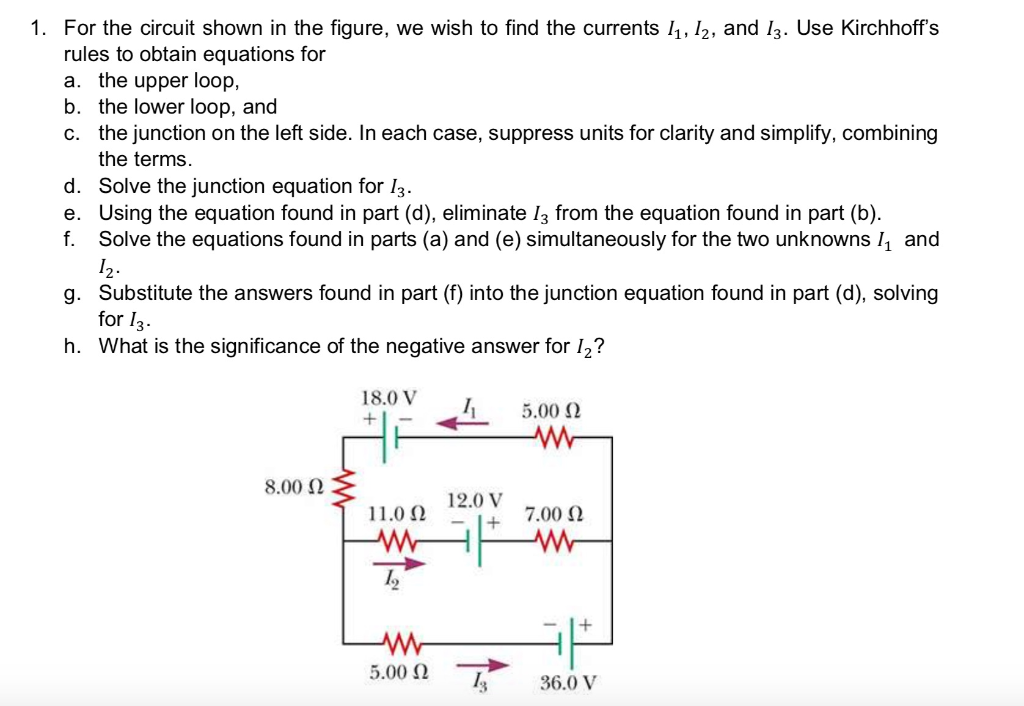1. For the circuit shown in the figure, we wish to find the currents 11, 12, and 13. Use Kirchhoff's rules to obtain equations for a. the upper loop, b. the lower loop, and c. the junction on the left side. In each case, suppress units for clarity and simplify, combining the terms. d. Solve the junction equation for 13. e. Using the equation found in part (d), eliminate 13 from the equation found in part (b). f. Solve the...

• ### I need the equations please!! Given the circuit below: A. Find all loop currents and voltage...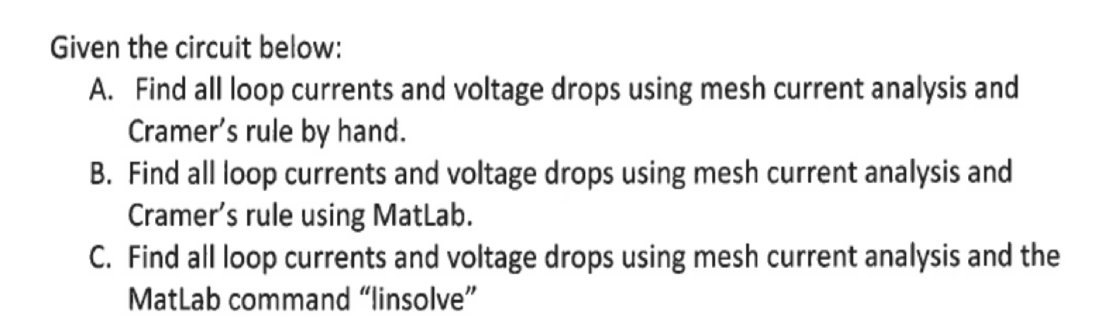I need the equations please!! Given the circuit below: A. Find all loop currents and voltage drops using mesh current analysis and Cramer's rule by hand. B. Find all loop currents and voltage drops using mesh current analysis and Cramer's rule using Matlab. C. Find all loop currents and voltage drops using mesh current analysis and the MatLab command "linsolve" 2

• ### A) For the schematic above find the state-space equations that define this system. B) Using the...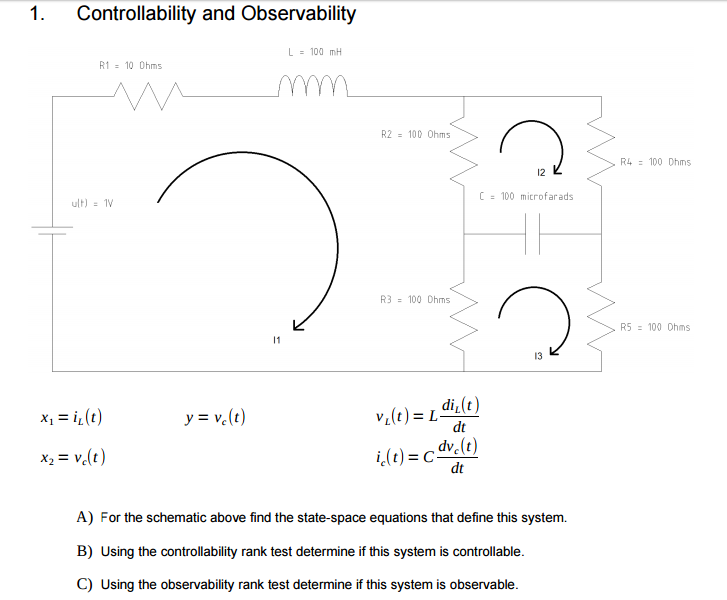A) For the schematic above find the state-space equations that define this system. B) Using the controllability rank test determine if this system is controllable. C) Using the observability rank test determine if this system is observable. 1. Controllability and Observability L = 100 m R1 = 10 Ohms Mm R2 = 100 Ohms R4 = 100 Ohms ( = 100 microfarads ult) 1V R3 = 100 Ohms R5 = 100 Ohms Xı = i(t) y = valt) vi(t) =...

• ### please help with equations 1, 2 and 3. I am confused! act. - approx 1.1001 act...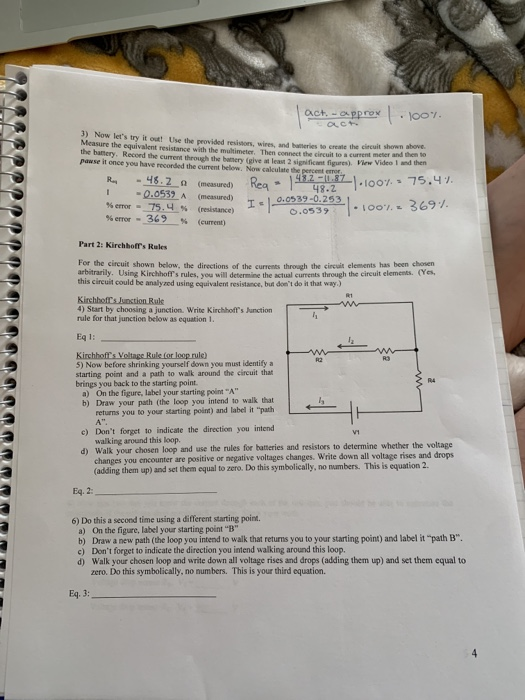please help with equations 1, 2 and 3. I am confused! act. - approx 1.1001 act 3) Now let's try it out! Use the provided resistors, wires, and bumseries to create the circuit shown above Measure the equivalent resistance with the multimeter. Then connect the circuito current meter den the battery. Record the current through the battery give a least 2 sificat) View Video and then powse it once you have recorded the current below. Now calculate the percent Ro...

• ### Please provide deep explaining of concepts, theories, and formulas that apply to each problem. I have...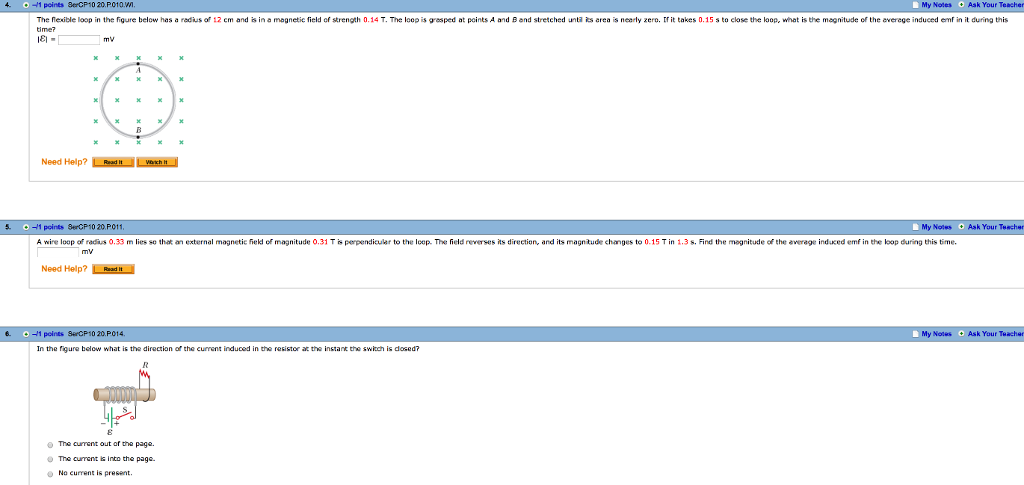Please provide deep explaining of concepts, theories, and formulas that apply to each problem. I have a physics final coming up and I need to be prepared, these are the practice questions. thank you. Please draw a sketches that will help me visualize the problem and help me solve it as well, thank you. 4. + -/1 points SerCP10 20.P010.vn. My Notes Ask Your Teacher The nexible loop in the figure below has a redius of 12 em and is...

• ### (PHYS 2212K students only) Using the variables in Equations (4), (5) and (6), use Kirchhoff's loo...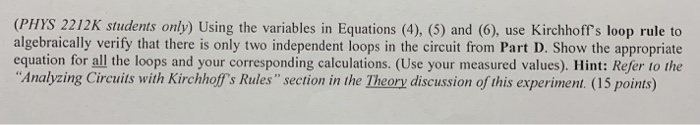see question below: equations 4,5 & 6: part D: my measured values: (PHYS 2212K students only) Using the variables in Equations (4), (5) and (6), use Kirchhoff's loop rule to algebraically verify that there is only two independent loops in the circuit from Part D. Show the appropriate equation for all the loops and your corresponding calculations. (Use your measured values). Hint: Refer to the Analyzing Circuits with Kirchhoffs Rules" section in the Theory discussion of this experiment. (15 points)...

Free Homework App